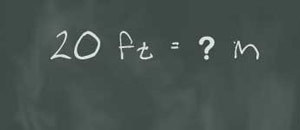# Feet To Meters

1 foot is equal to 0.3048 meters.1 meter is equal to 3.2808399 feet. To convert feet to meters you multiply the foot by 0.3048. To convert meters to feet, multiply the meter by 3.3.

### Examples

If a basketball player stands 1.9 meters, multiply that by 3.3. The result (6.27) is the height in feet. As stated you just need to multiply the feet by 0.3048 to get the figures in meters.
1 foot is equal to approximately .3 meters while 10 feet is equal to 3.5 meters.

20 feet is equivalent 6.10 meters, 30 ft to 9.14 meters and 40 ft 12.19 meters. 50 ft is equal to 15.24 ft and 60 ft is equal to 18.29 meters. Here are a few more feet to meter equivalents: 70 ft is the same as 21.34 ft, 80 ft is the same as 24.38 meters, 90 ft has a metric equivalent of 27.43 meters and 100 ft to 30.48 meters.

### The Feet

1 foot is equal to 12 inches. There are three feet in a single yard. The unit is not part of the SI (International Standards) measurements. However it is the unit of length used in the United States, the UK and other countries. The foot length was agreed upon in 1959. That year the yard was determined to be 0.9144 yards and this meant 1 foot was equal to 0.3048 meters.

If converting feet to meters, keep in mind that one foot is also equal to 304.8 millimeters. The most common symbol used is ft. Often, the foot is denoted by an apostrophe. For example, 6’ 2” means 6 feet two inches.

Before the agreement, the foot was measured in different ways. The Amsterdam foot was equal to 0.2831 m while the Danish foot was equal to 0.31385 m. The French used the pied du roi which was equal to 0.32484 meters.

Other old measurements included the Norwegian foot (0.31375 m), the Portuguese foot (0.3285 m), the Rotterdam foot (0.296 m) and the Swedish foot (0.2969 m). The need to convert feet to meters led to the elimination of these other measurements, including the Venetian foot (0.34773 m) and the Spanish foot (0.32483 m).

### Background

The feet had been used for measuring since ancient times. Some archaeologists suggested that it was first used in Sumer. However some have suggested that the measurement or a variation of it was first used in Egypt.

### The Meter

The meter is symbolized by m and is also equal to 1.094 yards. The meter was developed in 1790 by the French National Assembly. Originally it was said to be the distance between two marks of the platinum / iridium bar.

The measurement was set to equal one ten millionth of the distance from the Equator to the North Pole all the way to Paris. The measurement was later redefined to be the space traversed by light in 1/299,792,458 in a second.

By uncovering the ways to convert feet to meters, it will be easier to assess the various figures you come across when working or studying.